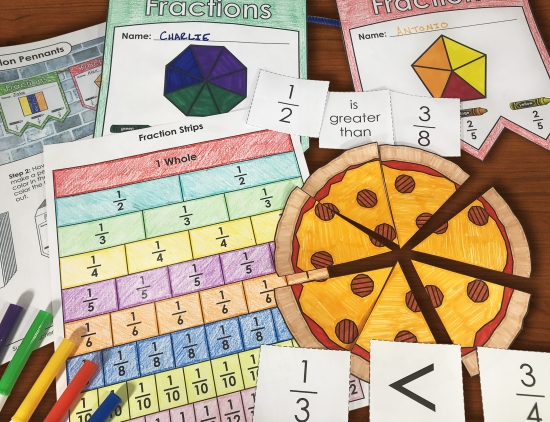# Fraction Worksheets for KidsBrowse the Super Teacher Worksheets fractions activities collection today! You'll find worksheets for popular fractions topics, including basic fractions, equivalent fractions, simplifying fractions, comparing fractions, ordering fractions, fractions of sets, mixed numbers, improper fractions, reciprocal fractions, adding fractions, subtracting fractions, multiplying fractions, dividing fraction, and more!